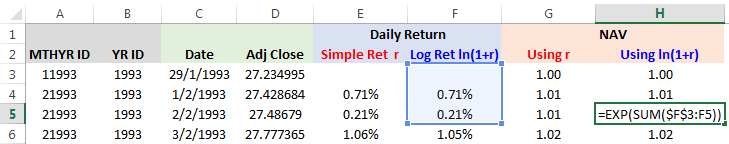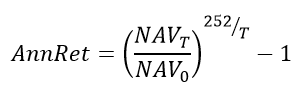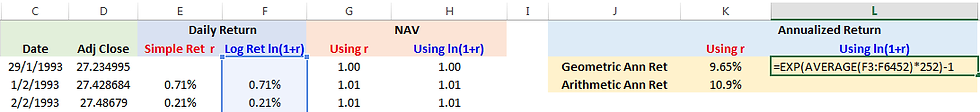top of pageSearch
• Eng Guan

# Magic of Log Returns: Practical – Part 2

Updated: Mar 10

This is Part 2 and the final part of the series on Log Returns. I will be focusing solely on its application here using Microsoft Excel. I am using the 2013 version. If you are using a different version, it is fine as well. While you might find some changes in the layout, the Excel built-in formulas I am using should be the same. For those who have no idea what log returns are about, you can take a look at my previous post on Part 1 Magic of Log Returns – Concept.

For this exercise, I am using the historical data for SPDR S&P 500 ETF – SPY from yahoo finance. Yahoo Finance is a very useful site for free End-of-Day (EOD) stock data. They use to support APIs that allow us to pull data directly into Excel. But unfortunately, they seemed to have terminated this service permanently after Verizon acquired Yahoo. Nonetheless, you can still download the data manually from the website.

This is the excel file to go with this post: Log-Returns-Part-2. I have retained only the ‘Date’ and ‘Adj Close’ of the SPY column here. The rest of the columns are not required. ‘Adj Close’, or in full Adjusted Close, are prices that account for dividends and share splits. Unless you have good reasons to exclude dividends and splits from your calculations, it is a good practice to make use of it.

I will show 4 simple applications of log returns here:

(1) Calculating daily simple and daily log returns.

(2) Constructing the NAV that starts at 1.

(3) Calculating annualized returns using both simple and log returns.

(4) Creating a performance table using log returns.

## 1. Calculating and Comparing Simple and Log Daily Returns

This is the most straightforward part. Open up the Excel file and go to sheet ‘Log & Simple Returns’. Then refer to columns E and F for the formula keyed into the cells. For simple returns, you can get them by dividing today’s price by yesterday’s and then subtracting 1 from the result.Then using the simple returns in Column E, you can compute your log returns. This is done by applying the Excel built-in formula for natural logarithm, ln() on the simple return. Nice and simple.You might observe that the daily log returns and simple returns are very close in value to each other. But that is not always the case. If the simple daily returns are very large, the difference can be significant. I show an example on this later.

## 2. Calculating NAV

NAV is really the same series as Adjusted Close, except that I have rebased the Adjusted Close to start at 1. It is more apparent from the NAV how much a security or portfolio has gained since its inception. For example, if the NAV today is 10, that means the value of the portfolio has grown from 1 to 10 since inception, or a 900% increase. If we talk in terms of adjusted close, then it is harder to see the performance without doing further calculations.

There are 2 ways you can go about building the NAV column.

• Using simple returns – Multiply the previous day’s NAV with today’s 1 + simple returns.• Using log returns – Sum up all prior log returns including today’s and then apply the exponential function on it to get the NAV.## 3. Annualized Returns

We always like to talk in terms of annual performance as people like to know how much they can expect to make a year in percentage terms. That is why in most of the fund reports, you will find a standard metric called annualized returns. It is also known as the Compound Annual Growth Rate (CAGR) or the Geometric Annual Return. And how do we calculate this in Excel?

• Using Simple Returns – We can make use of the NAV we computed earlier. You take the final NAV and divide it by the inception NAV (which by the way is a value of 1). The value is then raised to the power of 252/T where T is the number of trading days over the entire period considered. 252 is the number of US trading days in a year. The result is then subtracted by 1 to get the annualized return. To generalize it further, if we are using weekly data instead, then we will be raising it to the power of 52/T. There are 52 weeks in a year and T is now in terms of weeks. I believe you get the idea.• Using Log Returns – We multiply the average of the daily log returns over the period by 252 and then apply the exponential function to it. Then we subtract 1 from the result to get the annualized return. If we are working with weekly returns, then we multiply the average by 52, or if monthly, then by 12.As a word of caution, some people find it more convenient to just use the arithmetic average as a proxy for annualized returns. And what do I mean by that? Using our exercise as an example, they will use the average of the daily simple return series and multiply it by 252. But for reasons that I have already mentioned in Part 1, you should use the geometric return instead. The arithmetic approach can differ significantly if the return series is volatile. You can refer to the worksheet “Inflated Returns”. In this sheet, I have inflated the daily returns of SPY by 5 times. The CAGR or geometric annualized returns is 12.73%. But if you used an arithmetic average on the simple returns instead, you would end up with a whopping 54.4%.

## 4. Constructing A Performance Table

Performance table is a common sight in monthly reports. You may want to create one but find it too much of a pain to manually locate and find all the corresponding NAVs to compute the monthly returns. Actually, it does not have to be that difficult. All you need is to make use of log returns and a few functions in Excel.The performance table we want to create is segregated by month and year. So to get those figures, you will have to identify the returns by their corresponding month and year. We can do this by creating identifier columns that extract the month and year of each date using the MONTH() and YEAR() functions. In column A, we concatenate MONTH() & YEAR() to get a unique MTHYR ID that corresponds to each date to a specific month and year. This will assist us later in getting the monthly returns.Column B just gives us the Year. This is to help us in computing the yearly returns.Now, to get the returns for each specific month and year, we just need to sum up the log returns for the days where their IDs match that particular month and year. This can be done using the SUMIF function. You then apply the exponential function on the result and subtract 1 to back out the simple returns for the month. You can lookup the SUMIF function in Excel Help. They have a writeup and examples on how it can be used.The yearly returns are done in a similar way. You just need to add up the log returns of the days that have an ID that matches the specific year you are looking for using SUMIF.## End Of The Magic

That is all I have regarding log returns for now. There are other ways you can implement the things I have done here. For example, you can also write a VBA script to churn out a performance table. Feel free to explore other means of implementation. Meanwhile, hope you gained something out of this and have a good day.

Feel free to share the post if you find it useful.

Want to know more about AllQuant?